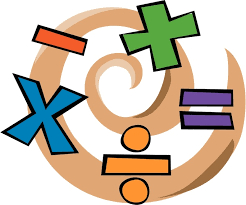# Find error in calculation

Sally's calculations are as follows 39-9×4+12
=30×16
=480

What are the errors in this calculation?

x =  15

### Step-by-step explanation:Did you find an error or inaccuracy? Feel free to write us. Thank you!

Showing 1 comment:
Dr Math
See our decimal calculator:

https://www.hackmath.net/en/calculator/decimal?input=39-9%C3%974%2B12

Sally has an error; she firstly subtracted and added and then multiplied operands. The math problem is about the order of operations without brackets. Multiply first, then add and subtract.